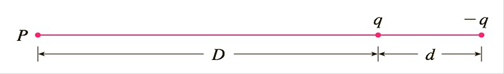Chapter 11.11, Problem 33E

Chapter
Section
Textbook Problem

An electric dipole consists of two electric charges of equal magnitude and opposite sign. If the charges are q and − q and are located at a distance d from each other, then the electric field E at the point P in the figure is E = q D 2 − q ( D + d ) 2 By expanding this expression for E as a series in powers of d / D show that E is approximately proportional to 1 / D 3 when P is far away from the dipole.To determine

To show:

E is approximately proportional to 1D3 when P is far away from dipole

Explanation

1) Concept:

The binomial series:

If k is any real number and x<1, then

1+xk=n=0knxn=1+kx+kk-12!x2+kk-1k-23!x3+

2) Given:

E=qD2-qD+d2

3) Calculation:

The electric field E at a point P given in the figure below is

E=qD2-qD+d2

To expand E as power of  dD rewrite  E as

E=qD2-qD+d2

=qD2-qD21+dD2

=qD21-1+dD-2

Use binomial series to expand 1+dD-2 as,

1+dD-2=1+-2dD+-2-2-12!dD2+-2-2-1-2-2

Still sussing out bartleby?

Check out a sample textbook solution.

See a sample solution

The Solution to Your Study Problems

Bartleby provides explanations to thousands of textbook problems written by our experts, many with advanced degrees!

Get Started

In Exercises 516, evaluate the given quantity. log1/212

Finite Mathematics and Applied Calculus (MindTap Course List)

In Problems 1-6, find the second derivative.

Mathematical Applications for the Management, Life, and Social Sciences

Find f'(a). f(t) = 2t3 + t

Single Variable Calculus: Early Transcendentals